• ## 递归算法时间复杂度分析

万次阅读 多人点赞 2018-09-17 16:16:59
递归算法时间复杂度分析 时间复杂度： 一般情况下，算法中基本操作重复的次数就是问题规模n的某个函数f（n），进而分析f（n）随n的变化情况并确定T（n）的数量级。这里用‘o’来表示数量级，给出算法时间复杂度。 T...
                             递归算法时间复杂度分析

时间复杂度：

一般情况下，算法中基本操作重复的次数就是问题规模n的某个函数f（n），进而分析f（n）随n的变化情况并确定T（n）的数量级。这里用‘o’来表示数量级，给出算法时间复杂度。
T（n）=o（f（n））；
它表示随问题规模n的增大，算法的执行时间增长率和f（n）增长率成正比，这称作算法的渐进时间复杂度。而我们一般情况下讨论的最坏的时间复杂度。
空间复杂度：
算法的空间复杂度并不是实际占用的空间，而是计算整个算法空间辅助空间单元的个数，与问题的规模没有关系。算法的空间复杂度S（n）定义为该算法所耗费空间的数量级。
S（n）=o（f（n））
若算法执行所需要的辅助空间相对于输入数据n而言是一个常数，则称这个算法空间复杂度辅助空间为o（1）；
递归算法空间复杂度：递归深度n*每次递归所要的辅助空间，如果每次递归所需要的辅助空间为常数，则递归空间复杂度o（n）。

递归算法分析

1、利用数列知识

累加法：递推关系式为an+1−an=f(n)an+1−an=f(n)采用累加法。
累乘法：递推关系式为an+1an=f(n)an+1an=f(n)采用累乘法。
构造法：递推关系式为(1)aa+1=pan+qaa+1=pan+q，(2)aa+1=pan+qnaa+1=pan+qn，都可以通过恒等变形，构造出等差或等比数列，利用等差或等比数列的定义进行解题，其中的构造方法可通过待定系数法来进行。
和化项法：递推公式为Sn=f(n)Sn=f(n)或Sn=f(an)Sn=f(an)一般利用
an={S1，Sn−Sn−1，当n=1当n>=2an={S1，当n=1Sn−Sn−1，当n>=2

用特征方程求解递推方程（感觉比较生僻，不做解释）
迭代法： 从原始递推方程开始，反复将对于递推方程左边的函数用右边的等式代入，直到得到初值，然后将所得的结果进行化简。
例如在调用归并排序mergeSort(a,0,n-1)对数组a[0...n−1]a[0...n−1]排序时，执行时间T(n)T(n)的递推关系式为：
T(n)={O(1)，2T(n2)+O(n)，当n=1当n>=2T(n)={O(1)，当n=12T(n2)+O(n)，当n>=2

其中，O(n)O(n)为merge()所需要的时间，设为cncn（c为正常量）。因此：
T(n)=2T(n2)+cn=2(2T(n4)+cn2)+cn=22T(n4)+2cn=23T(n8)+3cn=...=2kT(n2k)+kcn=nO(1)+cnlog2n=O(nlog2n),(假设n=2k,则k=log2n)T(n)=2T(n2)+cn=2(2T(n4)+cn2)+cn=22T(n4)+2cn=23T(n8)+3cn=...=2kT(n2k)+kcn=nO(1)+cnlog2⁡n=O(nlog2⁡n),(假设n=2k,则k=log2⁡n)

忽略求解细节。在我们求解递归式时，因为最终是要求得一个时间上限，所以在求解时常常省略一些细节。比如mergeSort(a,0,n-1)运行时间的实际递归式应该是：

T(n)={O(1)，T(⌈n2⌉)+T(⌊n2⌋)+O(n)，当n=1当n>=2T(n)={O(1)，当n=1T(⌈n2⌉)+T(⌊n2⌋)+O(n)，当n>=2

但我们忽略这些上取整、下取整以及边界条件，甚至假设问题规模n=2kn=2k，这都是为方便求解而忽略的细节。经验和一些定理告诉我们，这些细节不会影响算法时间复杂度的渐近界。

类似的，我们也可以用迭代法求解汉诺塔递归求解时的时间复杂度。但遗憾的是，迭代法一般适用于一阶的递推方程。对于二阶及以上（即T(n)依赖它前面更多个递归项T(n)依赖它前面更多个递归项）的递推方程，迭代法将导致迭代后的项太多，从而使得求和公式过于复杂，因此需要将递推方程化简，利用差消法等技巧将高阶递推方程化为一阶递推方程。如在求快速排序算法平均时间复杂度T(n)T(n)的递推方程，T(n)T(n)依赖T(n−1)、T(n−2)、...、T(1)T(n−1)、T(n−2)、...、T(1)等所有的项，这样的递推方程也称为全部历史递推方程。（这里省略快速排序算法平均复杂度T(n)的求解过程）

小结：上面6种递推关系是高中、本科知识，在此重点介绍了迭代法，其它几种方法虽未在本篇中使用，但可以加深对递推式求解的认识。

2、代入法

代入法实质上就是数学归纳法，因此求递推式分为两步：

猜测解的形式；
用数学归纳法求出解中的常数，并证明解是正确的。
遗憾的是并不存在通用的方法来猜测递归式的正确解，需要凭借经验，偶尔还需要创造力。即使猜出了递归式解的渐近界，也有可能在数学归纳证明时莫名其妙的失败。正是由于该方法技术细节较为难掌握，因此这个方法不适合用来求解递归方程，反而比较适合作为其他方法检验手段。在此不做总结。可以翻阅《算法导论》进行学习。

3、递归树

递归树是一棵结点带权值的树。初始的递归树只有一个结点，它的权标记为T(n)T(n)；然后按照递归树的迭代规则不断进行迭代，每迭代一次递归树就增加一层，直到树中不再含有权值为函数的结点（即叶结点都为T(1)T(1)）。下面以递归方程

T(n)={O(1)，2T(n2)+O(n)，当n=1当n>=2;(假设n=2k,则k=log2n)T(n)={O(1)，当n=12T(n2)+O(n)，当n>=2;(假设n=2k,则k=log2⁡n)

来讲述递归树的迭代规则。

第一步： 把根结点T(n)T(n)用根是cncn、左结点为T(n2)T(n2)、右结点为T(n2)T(n2)的子树代替（即：以分解、合并子问题需要的代价为根，分解得到的子问题为叶的子树。其中常量c代表求解规模为1的问题所需的时间）；（如下如(a)→(b)(a)→(b)）
第二步：把叶结点按照“第一步”的方式展开；T(n2)T(n2)用根是cn/2cn/2、左节点为T(n4)T(n4)、右结点为T(n4)T(n4)的子树代替。（如下如(b)→(c)(b)→(c)）
第三步：反复按照“第一步”的方式迭代，每迭代一次递归树就增加一层，直到树中不再含有权值为函数的结点（即叶结点都为T(1)T(1)）。（如下如(c)→(d)(c)→(d)）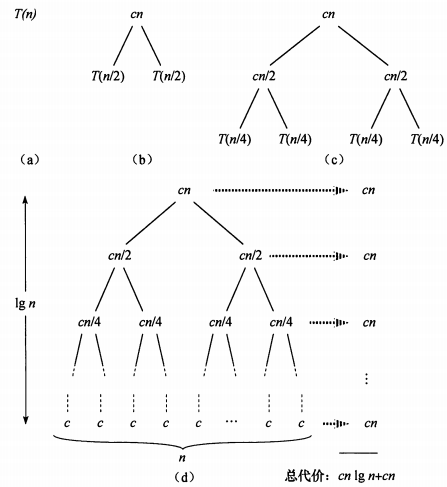在得到递归树后，将树中每层中的代价求和，得到每层代价，然后将所有层的代价求和，得到所有层次的递归调用的总代价。在上图(d)部分中，完全展开的递归树高度为lgnlg⁡n(树高为根结点到叶结点最长简单路径上边的数目)，所有递归树具有lgn+1lg⁡n+1层，所以总代价为cn∗(lgn+1)cn∗(lg⁡n+1)，所有时间复杂度为Θ(nlgn)Θ(nlg⁡n)。

总结：递归树模型求解递归方程，本质上就是迭代思想的应用，利用递归方程迭代展开过程构造对应的递归树，然后把每层的时间代价进行求和。不过递归树模型更直观，同时递归树也克服了二阶及更高阶递推方程不方便迭代展开的痛点。

4、主方法求解递推式

主方法为如下形式的递归式提供了一种“菜谱”式的求解方法，如下所示

T(n)=aT(n/b)+f(n)T(n)=aT(n/b)+f(n)

其中a≥1a≥1和b>1b>1是常数，f(n)f(n)是渐近正函数。这个递推式将规模为n的问题分解为a个子问题，每个子问题的规模为n/bn/b，a个子问题递归地求解，每个花费时间T(n/b)T(n/b)。函数f(n)f(n)包含了问题分解和子问题解合并的代价。同样，这个递归式也没有考虑上取整、下取整、边界条件等，结果不会影响递归式的渐近性质。

定理4.1(主定理) 令a≥1和b>1是常数，f(n)f(n)是一个函数，T(n)T(n)是定义在非负整数上的递归式：

T(n)=aT(n/b)+f(n)T(n)=aT(n/b)+f(n)

其中我们将n/bn/b解释为⌊n/b⌋⌊n/b⌋或⌈n/b⌉⌈n/b⌉。那么T(n)T(n)有如下渐近界：
1. 若对某个常数ε>0ε>0有f(n)=O(n(logba)−ε)f(n)=O(n(logb⁡a)−ε)，则T(n)=Θ(nlogba)T(n)=Θ(nlogb⁡a)
2. 若f(n)=Θ(nlogba)f(n)=Θ(nlogb⁡a)，则T(n)=Θ(nlogbalgn)T(n)=Θ(nlogb⁡alg⁡n)。
3. 若对某个常数ε>0ε>0有f(n)=Ω(n(logba)+ε)f(n)=Ω(n(logb⁡a)+ε)，且对某个常数c<1c<1和所有足够大的n有af(n/b)≤cf(n)af(n/b)≤cf(n)，则T(n)=Θ(f(n))T(n)=Θ(f(n))

在使用主定理之前，要比较f(n)和(nlogba)f(n)和(nlogb⁡a)的大小，这个大小不是算术意义上的大小比较，而是要在多项式意义上比较。以上三种情况在多项式意义上并未覆盖f(n)f(n)的所有可能性。情况1和情况2之间有一定间隙；情况2和情况3之间也有一定间隙。如果f(n)落在这两个间隙中，或者情况3中 正则条件不成立，就不能使用主方法来求递归式。
如在递归式：T(n)=2T(n/2)+nlgnT(n)=2T(n/2)+nlg⁡n中，因为 nlogba=n<f(n)=nlgnnlogb⁡a=n<f(n)=nlg⁡n，但是f(n)f(n)并不大于n一个多项式因子nεnε ,因为对于给定的ε>0ε>0当n足够大时，均有nε>lgnnε>lg⁡n。所以找不到这样 ε>0ε>0，该递归式落入了情况2和情况3之间的间隙，不能使用主定理。
最后给出主定理应用的几个练习题：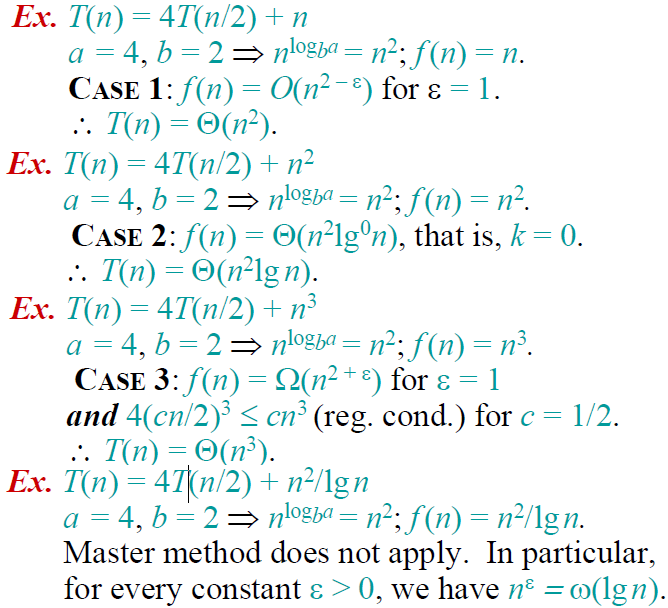具体举例分析：

【代入法】代入法首先要对这个问题的时间复杂度做出预测，然后将预测带入原来的递归方程，如果没有出现矛盾，则是可能的解，最后用数学归纳法证明。

【举   例】我们有如下的递归问题：T(n)=4T(n/2)+O(n)，我们首先预测时间复杂度为O(n2),不妨设T(n)=kn2（其中k为常数），将该结果带入方程中可得：左=kn2，右=4k(n/2)2+O(n)=kn2+O(n),由于n2的阶高于n的阶，因而左右两边是相等的，接下来用数学归纳法进行验证即可。

【迭代法】迭代法就是迭代的展开方程的右边，直到没有可以迭代的项为止，这时通过对右边的和进行估算来估计方程的解。比较适用于分治问题的求解，为方便讨论起见，给出其递归方程的一般形式：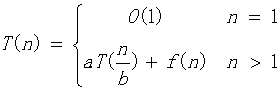【举   例】下面我们以一个简单的例子来说明：T(n)=2T(n/2)+n2,迭代过程如下：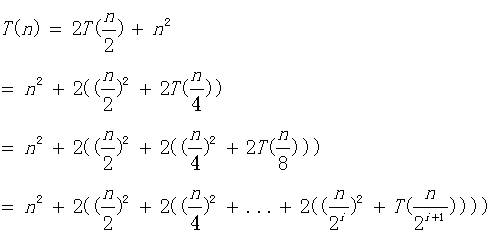容易知道，直到n/2^(i+1)=1时，递归过程结束，这时我们计算如下：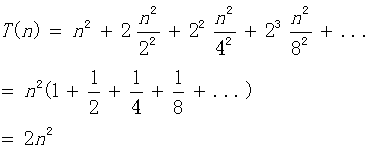到这里我们知道该算法的时间复杂度为O(n2)，上面的计算中，我们可以直接使用无穷等比数列的公式，不用考虑项数i的约束，实际上这两种方法计算的结果是完全等价的，有兴趣的同学可以自行验证。

【公式法】这个方法针对形如：T(n) = aT(n/b) + f(n)的递归方程。这种递归方程是分治法的时间复杂性所满足的递归关系，即一个规模为n的问题被分成规模均为n/b的a个子问题，递归地求解这a个子问题，然后通过对这a个子问题的解的综合，得到原问题的解。这种方法是对于分治问题最好的解法，我们先给出如下的公式：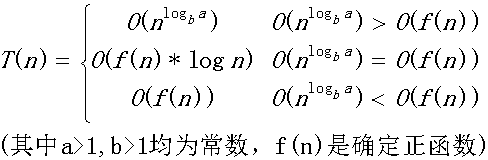公式记忆：我们实际上是比较n^logba和f(n)的阶，如果他们不等，那么T(n)取他们中的较大者，如果他们的阶相等，那么我们就将他们的任意一个乘以logn就可以了。按照这个公式，我们可以计算【迭代法】中提到的例子：O(f(n))=O(n2),容易计算另外一个的阶是O(n),他们不等，所以取较大的阶O(n2)。太简单了，不是吗？

需要注意：上面的公式并不包含所有的情况，比如第一种和第二种情况之间并不包含下面这种情况：f(n)是小于前者，但是并不是多项式的小于前者。同样后两种的情况也并不包含所有的情况。为了好理解与运用的情况下，笔者将公式表述成如上的情况，但是并不是很严谨，关于该公式的严密讨论，请看这里。但是公式的不包含的情况，我们很少很少碰到，上面的公式适用范围很广泛的。

特别地，对于我们经常碰到的，当f(n)=0时，我们有：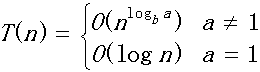【母函数法】母函数是用于对应于一个无穷序列的幂级数。这里我们解决的递归问题是形如：T(n)=c1T(n-1)+c2T(n-2)+c3T(n-3)+...+ckT(n-k)+f(n)。为说明问题简便起见，我们选择斐波那契数列的时间复杂度作为例子进行讨论。

【举  例】斐波那契数列递归公式：T(n)=T(n-1)+T(n-2)。这里我们假设F(n)为第n项的运算量。则容易得到：F(n)=F(n-1)+F(n-2),其中F(1)=F(2)=1.我们构造如下的母函数：G(x)=F(1)x+F(2)x2+F(3)x3+......,我们可以推导如下：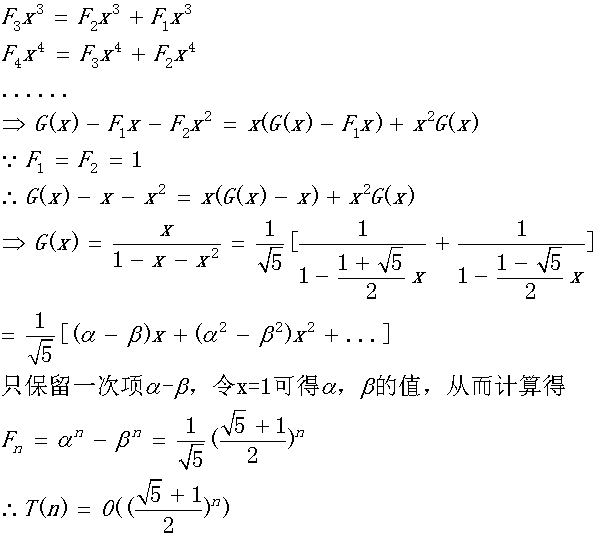上面的方法计算相对来说是比较简单的，关键在于对于母函数的理解，刚开始的时候可能不是很好理解，对于母函数可以参考这里和维基百科这里。

【差分方程法】可以将某些递归方程看成差分方程，通过解差分方程的方法来解递归方程，然后对解作出渐近阶估计。这里我们只考虑最长常见的递归形式，形如：T(n)=c1T(n-1)+c2T(n-2)+c3T(n-3)+...+ckT(n-k)+f(n)，其中c1,c2,...ck为常数且不等于0；我们对该方程的求解如下：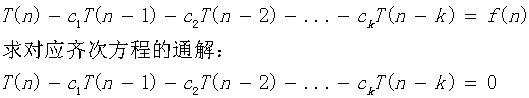对应上面的齐次方程的特征方程为：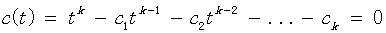如果解得t=r是该特征方程的m重根，则这m个解的形式为：{rn     n*rn      n2rn   ...    nm-1rn},其余的关于复数解的形式和普通的线性方程组的形式类似，不再赘述。接下来，我们要求解该方程的对应非齐次方程组的通解，这里我们针对该方程的特殊形式，不加证明地给出如下的通解形式：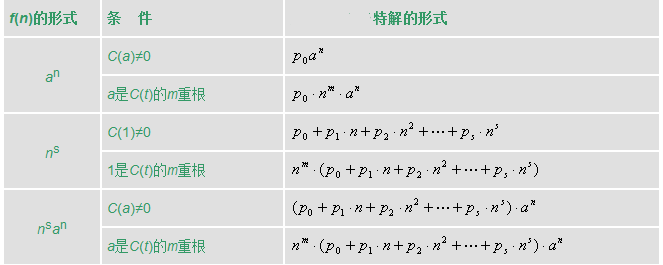则和线性代数中的解一样，原方程的解等于齐次方程组的通解+特解，即：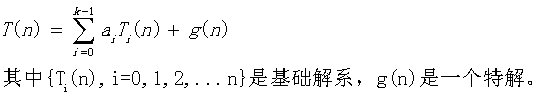最后由初始条件确定a(i)的值即可。

为了帮助理解，我们举两个例子看看，就明白是怎么回事了。

【举 例1】递归方程如下：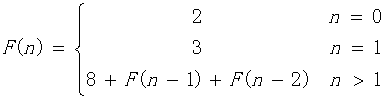(1)写出对应齐次方程的特征方程：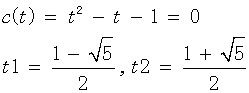得到基础解系为：{t1n,  t2n}

(2)计算特解，对于本题，直接观察得特解为：-8

(3)得到原方程解的形式为：T(n)=a0t1n+a1t2n-8

(4)代入n=0，n=1的情况，得到a0,a1，最后可得: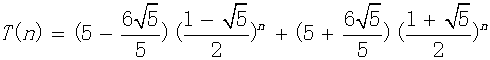可以看到该方程形式和上面讨论过的斐波那契数列只差一个常数8，因而两者的时间复杂度是相同的。有兴趣的同学可以按照这个方法再次计算斐波那契数列的时间复杂度验证一下。

【举  例2】递归方程如下：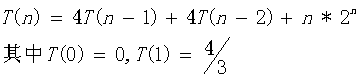（1）计算对应齐次方程的基础解析：特征方程为：C(t)=t^2-4t-4=0,得到一个2重根t=2.因而其基础解为：{2n      n*2n}

（2）由于f(n)=n*2n，对应上面表格的最后一种情况，得到特解形式为：T(n)=n2(p0+p1n)2n代入原递归方程可得：p0=1/2,p1=1/6

（3）原方程解的形式为：T(n)=a0*2n+a1*n*2n+n2(1/2+n/6)2n,代入T(0),T(1)得：a0=a1=0

（4）综上：T(n)=n2(1/2+n/6)2n

因而时间复杂度为：O(n32n).

参考：https://blog.csdn.net/so_geili/article/details/53444816

https://blog.csdn.net/m0_37734999/article/details/78751882

https://www.cnblogs.com/hlongch/p/5749038.html

展开全文• 递归次数过多容易造成栈溢出等，所以一般不提倡用递归算法设计程序。 递归算法有个很重要的就是递归算法的出口条件，满足出口条件，就不递归了。 举个栗子：代码如下： class Factorial { //递归函数 ...
递归算法是方法内调用自身；
递归算法必须要有个明确的条件作为算法结束的出口，被称为递归出口；
递归算法的不足：在递归调用的过程中系统为每一层的返回点、局部量等开辟了栈来存储。递归次数过多容易造成栈溢出等，所以一般不提倡用递归算法设计程序。
递归算法有个很重要的就是递归算法的出口条件，满足出口条件，就不递归了。
举个栗子：代码如下：
class Factorial {    //递归函数    int fact(int n){        if(n==1){            return 1;        }else{            return fact(n-1)*n;        }    }}public class TestFactorial {    public static void main(String[] args) {        Factorial factorial= new Factorial();        System.out.println("factorial(10)="+factorial.fact(10));    }}结果截图：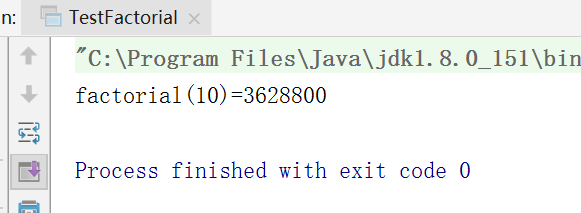再举个栗子：这是个移动盘子的实验
public class Hanio {    public static void main(String[] args){        int i=3;        char a ='A',b='B',c='C';        hanio(i,a,b,c);    }    public static void hanio(int n,char a,char b,char c){        if(n==1)            System.out.println("移动"+n+"号盘子从"+a+"到"+c);        else{            hanio(n-1,a,c,b);//把上面n-1个盘子从a借助b搬到c            System.out.println("移动"+n+"号盘子从"+a+"到"+c);//紧接着直接把n搬动c            hanio(n-1,b,a,c);//再把b上的n-1个盘子借助a搬到c        }    }}截图：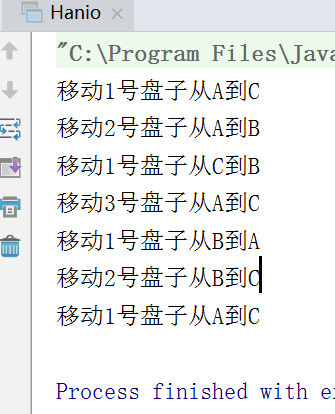转载于:https://www.cnblogs.com/GGboy-wu/p/9652269.html
展开全文• 递归效率高；递归代码写出来思路清晰，可读性强。 生成可执行文件大小应该...递归的话函数调用是有开销的，而且递归次数受堆栈大小的限制。 以二叉树搜索为例： bool search(btree* p, int v) { if (null ...
转载自：https://blog.csdn.net/mhsszm/article/details/78445591

非递归效率高；递归代码写出来思路清晰，可读性强。

生成可执行文件大小应该和编译器有关吧。。。。

递归的话函数调用是有开销的，而且递归的次数受堆栈大小的限制。
以二叉树搜索为例：

bool search(btree* p, int v)
{
if (null == p)
return false;

if (v == p->v)
return true
else
{
if (v < p->v)
return search(p->left, v);
else
return search(p->right, v);
}
}

如果这个二叉树很庞大，反复递归函数调用开销就很大，万一堆栈溢出怎么办？
现在我们用循环改写：

bool search(btree* p, int v)
{
while (p)
{
if (v == p->v)
return true;
else
{
if (v < p->v)
p = p->left;
else
p = p->right;
}
}
return false;
}

递归好处：代码更简洁清晰，可读性更好

递归可读性好这一点，对于初学者可能会反对。实际上递归的代码更清晰，但是从学习的角度要理解递归真正发生的什么，是如何调用的，调用层次和路线，调用堆栈中保存了什么，可能是不容易。但是不可否认递归的代码更简洁。一般来说，一个人可能很容易的写出前中后序的二叉树遍历的递归算法，要写出相应的非递归算法就比较考验水平了，恐怕至少一半的人搞不定。所以说递归代码更简洁明了。

递归坏处：由于递归需要系统堆栈，所以空间消耗要比非递归代码要大很多。而且，如果递归深度太大，可能系统撑不住。

楼上的有人说：
小的简单的用循环,,
太复杂了就递归吧,,免得循环看不懂
话虽然简单，其实非常有道理：对于小东西，能用循环干嘛要折腾？如果比较复杂，在系统撑的住的情况下，写递归有利于代码的维护（可读性好）。
另：一般尾递归（即最后一句话进行递归）和单向递归（函数中只有一个递归调用地方）都可以用循环来避免递归，更复杂的情况则要引入栈来进行压栈出栈来改造成非递归，这个栈不一定要严格引入栈数据结构，只需要有这样的思路，用数组什么的就可以。

至于教科书上喜欢n!的示例，我想只是便于递归思路的引进和建立。真正做代码不可能的。

循环方法比递归方法快, 因为循环避免了一系列函数调用和返回中所涉及到的参数传递和返回值的额外开销。

递归和循环之间的选择。一般情况下, 当循环方法比较容易找到时, 你应该避免使用递归。这在问题可以按照一个递推关系式来描述时, 是时常遇到的, 比如阶乘问题就是这种情况。反过来, 当很难建立一个循环方法时, 递归就是很好的方法。实际上, 在某些情形下, 递归方法总是显而易见的, 而循环方法却相当难找到。当某些问题的底层数据结构本身就是递归时, 则递归也就是最好的方法了。

递归其实是方便了程序员难为了机器。它只要得到数学公式就能很方便的写出程序。优点就是易理解，容易编程。但递归是用栈机制实现的（c++），每深入一层，都要占去一块栈数据区域，对嵌套层数深的一些算法，递归会力不从心，空间上会以内存崩溃而告终，而且递归也带来了大量的函数调用，这也有许多额外的时间开销。所以在深度大时，它的时空性就不好了。

循环其缺点就是不容易理解，编写复杂问题时困难。优点是效率高。运行时间只因循环次数增加而增加，没什么额外开销。空间上没有什么增加。

递归算法与迭代算法的设计思路区别在于：函数或算法是否具备收敛性，当且仅当一个算法存在预期的收敛效果时，采用递归算法才是可行的，否则，就不能使用递归算法。

当然，从理论上说，所有的递归函数都可以转换为迭代函数，反之亦然，然而代价通常都是比较高的。

但从算法结构来说，递归声明的结构并不总能够转换为迭代结构，原因在于结构的引申本身属于递归的概念，用迭代的方法在设计初期根本无法实现，这就像动多态的东西并不总是可以用静多态的方法实现一样。这也是为什么在结构设计时，通常采用递归的方式而不是采用迭代的方式的原因，一个极典型的例子类似于链表，使用递归定义及其简单，但对于内存定义(数组方式)其定义及调用处理说明就变得很晦涩，尤其是在遇到环链、图、网格等问题时，使用迭代方式从描述到实现上都变得很不现实。

把递归函数转换成非递归程序的一般方法

把递归算法转化为非递归算法有如下三种基本方法：

(1). 通过分析，跳过分解过程，直接用循环结构的算法实现求解过程。

(2). 自己用栈模拟系统的运行时栈，通过分析只保存必须保存的信息，从而用非递归算法替代递归算法。

(3). 利用栈保存参数，由于栈的后进先出特性吻合递归算法的执行过程，因而可以用非递归算法替代递归算法。

●      递归函数的原理
用栈保存未完成的工作，在适当的时候从栈中取出并执行。系统保存了工作的数据和状态，数据就是函数的局部变量，
状态就是程序指针。

●      非递归程序原理
1. 和递归函数的原理相同，只不过是把由系统负责保存工作信息变为程序自己保存，这样能减少保存数据的冗余(主要是
节省了局部变量的空间)，提高存储效率。

2. 把程序要完成的工作分成两类：手头工作和保存在栈中的待完成的工作。手头工作指程序正在做的工作。由于某些工作
不能一步完成，必须暂缓完成，于是可把它保存在栈中，这就是待完成的工作。

3. 手头工作必须有其结束条件，不能永远做下去；保存的待完成工作必须含有完成该项工作的所有必要信息。

4. 程序必须有秩序地完成各项工作。如，可把手头工作恰当处理（直接处理或暂时保存）后，才能继续接手下一步的工作。

5. 待完成工作必须转换成手头工作才能处理。

●      栈的大小
所有递归问题，其递归过程可以展开成一棵树，叶子节点是可解的，按照问题的要求，处理所有叶子节点，就可解决
问题本身。可能需要保存(Data, Status)，Data是工作数据，Status是工作状态；（Data, Status）决定了整个工作。
栈的大小等于树的高度-1，-1是因为根节点不需保存。

●      举例
例1.    汉诺塔问题
递归函数：

void Hanoi(UINT x, UINT y, UINT n)
// x    Source
// y    Destination
// n    Number of plates
{
if (n == 0) return;
Hanoi(x, x^y, n-1);
Move(x, y);
Hanoi(x^y, y, n-1);
} 

说明：x、y可取1、2、3三数之一，并且x≠y，x^y表示x、y按位异或，得到除x、y之外的第三个数。1^2=3, 1^3=2, 2^3=1

非递归程序：

#define N 5
tyepdef struct _HANOIDATA
{
UINT x;
UINT y;
UINT n;
}HANOIDATA;
void Hanoi(HANOIDATA hanoiData)
{
HANOIDATA  stack[N];
int        top = -1;      //stack pointer

while (hanoiData.n || top != -1)    // 存在手头工作或待完成工作
{
while (hanoiData.n)    // 处理手头工作直到无现成的手头工作，
// 即下次的手头工作必须从栈中取得
{
hanoiData.n --;
stack[++top] = hanoiData;  // 保存待完成工作
hanoiData.y ^= hanoiData.x; // 新的手头工作
}
if (top != -1)  // 存在待完成工作
{
hanoiData = stack[top--];  // 从栈中取出
Move(hanoiData.x, hanoiData.y);   // 直接处理
hanoiData.x ^= hanoiData.y; // 未处理完的转换成手头工作
}
}
} 

例2． 后根序遍历二叉树
递归函数：

void PostTraverse(BINARYTREE root)
{
if (root == NULL) return;
PostTraverse(root->LChild);
PostTraverse(root->RChild);
Visit(root);
}

非递归程序：

void PostTraverse(BINARYTREE p)
{
while ( p != NULL || !Stack.IsEmpty() )// 存在工作(手头或待完成)
{
while (p != NULL)    // 处理手头工作，直到无现成手头工作
{
Stack.Push(p, RCHILD_AND_ITSELF);
p = p->LChild;
}
if (!Stack.IsEmpty())  // 是否存在待完成工作
{
Stack.Pop(p, Tag);
if (Tag == RCHILD_AND_ITSELF)   // 情况一: RChild &Itself
{
Stack.Push(p,ONLY_ITSELF)  // 保存待完成工作
p = p->RChild; // 新的手头工作
}
else        //tag == ONLY_ITSELF,  情况二: Only Itself
{
visit(p);
p = NULL;     // 已无现成的手头工作
}
}
}
} 

●      总结
非递归程序的设计应注意：
1.      保存暂缓执行的工作
2.      无现成手头工作的条件
3.      无待完成工作的条件

程序模式

void NonRecursiveFunction(DATATYPE Data)
{
while ( ExistHandyWork() || ExistSavedWork() )
{
while ( ExistHandyWork() )
{

Process(Work, Status)  //Probably push work onto stack
NewHandyWork();
}
if ( ExistSavedWork() )
{
Pop(Work, Status);
Process(Work, Status);  //Probably generate new handy work
}
}
}

递归算法向非递归算法转换

递归算法实际上是一种分而治之的方法，它把复杂问题分解为简单问题来求解。对于某些复杂问题(例如hanio塔问题)，递归算法是一种自然且合乎逻辑的解决问题的方式，但是递归算法的执行效率通常比较差。因此，在求解某些问题时，常采用递归算法来分析问题，用非递归算法来求解问题；另外，有些程序设计语言不支持递归，这就需要把递归算法转换为非递归算法。

将递归算法转换为非递归算法有两种方法，一种是直接求值，不需要回溯；另一种是不能直接求值，需要回溯。前者使用一些变量保存中间结果，称为直接转换法；后者使用栈保存中间结果，称为间接转换法，下面分别讨论这两种方法。

1. 直接转换法

直接转换法通常用来消除尾递归和单向递归，将递归结构用循环结构来替代。

尾递归是指在递归算法中，递归调用语句只有一个，而且是处在算法的最后。例如求阶乘的递归算法：

long fact(int n)
{
if(n==0)
return 1;
else
return n*fact(n-1);
}

当递归调用返回时，是返回到上一层递归调用的下一条语句，而这个返回位置正好是算法的结束处，所以，不必利用栈来保存返回信息。对于尾递归形式的递归算法，可以利用循环结构来替代。例如求阶乘的递归算法可以写成如下循环结构的非递归算法：

long fact(int n)
{
int s=0;
for(int i=1; i<=n;i++)
s=s*i;//用s保存中间结果
return s;
}

单向递归是指递归算法中虽然有多处递归调用语句，但各递归调用语句的参数之间没有关系，并且这些递归调用语句都处在递归算法的最后。显然，尾递归是单向递归的特例。例如求斐波那契数列的递归算法如下：

int f(int n)
{
if (n==1 | | n= =0)
return 1;
else
return f(n-1)+f(n-2);

}

对于单向递归，可以设置一些变量保存中间结构，将递归结构用循环结构来替代。例如求斐波那契数列的算法中用s1和s2保存中间的计算结果，非递归函数如下：

int f(int n)
{
int i,s;
int s1=1, s2=1;
for(i=3; i<=n; ++i)
{
s=s1+s2;
s2=s1; // 保存f(n-2)的值
s1=s; //保存f(n-1)的值
}
return s;
}

2. 间接转换法

该方法使用栈保存中间结果，一般需根据递归函数在执行过程中栈的变化得到。其一般过程如下：

将初始状态s0进栈
while (栈不为空)
{
退栈，将栈顶元素赋给s;
if (s是要找的结果) 返回;
else
{
寻找到s的相关状态s1;
将s1进栈
}
}

间接转换法在数据结构中有较多实例，如二叉树遍历算法的非递归实现、图的深度优先遍历算法的非递归实现等等。

使用非递归方式实现递归问题的算法程序,不仅可以节省存储空间,而且可以极大地提高算法程序的执行效率。本文将递归问题分成简单递归问题和复杂递归问题;简单递归问题的非递归实现采用递推技术加以求解,复杂递归问题则根据问题求解的特点采用两类非递归实现算法,使用栈加以实现。

展开全文• 递归算法通常很简洁，但递归算法解题的运行效率较低，一般不提倡这种算法 4.在递归调用的过程中系统为每一层的返回点，局部量等开辟栈来存储。递归次数过多容易造成栈溢出等。所以一般不提倡用...
一.什么是递归算法？    递归算法是一种直接或者间接调用自身的算法的过程。二.递归的特点？   1.递归就是在过程或者函数里调用自身。   2. 在使用递归过程中必须有个明确的递归结束条件，成为递归出口   3.递归算法通常很简洁，但递归算法解题的运行效率较低，一般不提倡这种算法   4.在递归调用的过程中系统为每一层的返回点，局部量等开辟栈来存储。递归次数过多容易造成栈溢出等。所以一般不提倡用递归算法设计程序。三.递归的设计  一个递归调用可以导致更多的递归调用，因为这个方法继续吧每个子问题分解成新的子问题，直到达到一个终止条件。当问题大道这个终止条件时候将结果返回给调用者。然后调用者进行计算并将结果返回给自己的调用者。这个过程持续，一级一级进行，直到结果传给原始的调用者为止。1.递归的定义　递归定义就是对问题分解，将一个问题分解为规模较小的问题并用相同的程序去解决。递归方法实际体现了“以此类推”“用同样的步骤重复”等思想，有点类似数学里的找规律推理出来的递推公式（函数）2.递归的终止条件：跳出递推，返回最小问题的解 得出最小问题的解，返回给调用者经典例子：1.递归求和 1+2+3+4+...+npublic static Integer recursionSum(Integer n){
if(n>0){
return n+recursionSum(n-1);
}else{
return 0;
}
}2.2.递归阶乘n! = n * (n-1) * (n-2) * ...* 1(n>0)public static Integer recursionMulity(Integer n){
if(n==1){
return 1;
}
return n*recursionMulity(n-1);
}3.河内塔问题内塔问题/**
* @param n 汉诺塔的层数
* @param x x柱 起点柱(A)
* @param y y柱 目标柱(B)
* @param z z柱 中转柱(C)
* 其中 A B C 只是作为辅助思考
*/
public int hanoiCount(int n, char x ,char y ,char z){
int moveCount=0;
//H(0)=0
if (n==0){
//什么也不做
return 0;
}else {
//递推公式：H(n)=H(n-1) + 1 + H(n-1)
//将n-1个圆盘从x移动到z,y为中转柱
moveCount += hanoiCount(n-1,x,z,y); //------------->解出n-1层汉诺塔:H(n-1)

//移动最大圆盘到目的柱
moveCount += 1; //---------------------------------> 1

//将n-1个圆盘从z移动到y,x为中转柱
moveCount +=hanoiCount(n-1,z,y,x);//--------------->解出n-1层汉诺塔:H(n-1)
}

return moveCount;
}4.斐波那契数列中的递归思想假如动物中有一种特殊的种类，它出生2天后就开始以每天1只的速度繁殖后代。假设第1天，有1只这样的动物（该动物刚出生，从第3天开始繁殖后代）。那么到第11天，共有多少只呢？我们先来按一般顺序思考，先不要考虑第11天，先从第1天开始，看能不能找出规律：
【第1天】只有1只动物
【第2天】只有1只动物，还没有繁殖后代，总量为1
【第3天】第1天的1只动物，繁殖1个后代，总量为2
【第4天】第1天的1只动物又繁殖1只，其他还没繁殖，总量为3
【第5天】第1天和第3天出生的动物又繁殖1个后代，其他没有繁殖，总量为5
【第n天】.....

第1天 ------1
第2天 ------1
第3天 ------2 = 1 + 1
第4天 ------3 = 1 + 2
第5天 ------5 = 2 + 3
第6天 ------8 = 3 + 5
第7天 ------13 = 5 + 8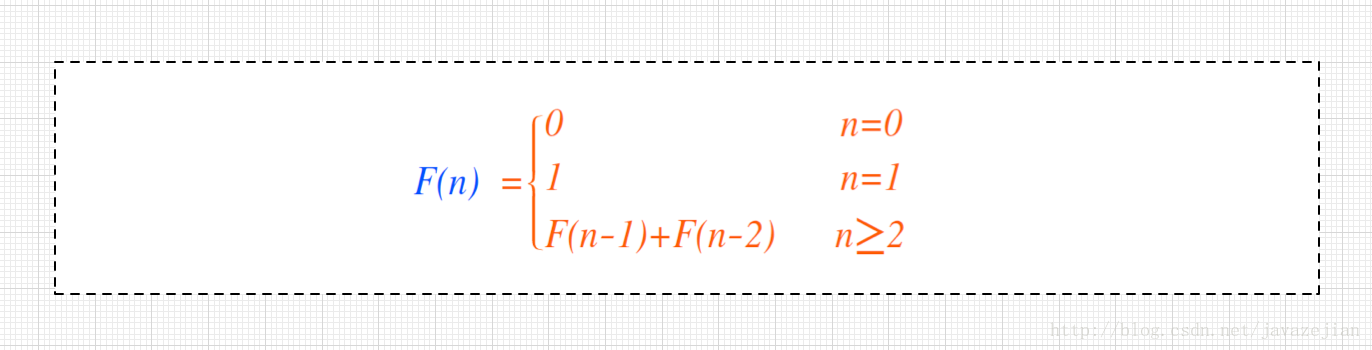/**
* 斐波那契数列的实现
* 0,1,1,2,3,5,8,13,21......
* @param day
*/
public long fibonacci(int day){

if(day==0){ //F(0)=0
return 0;
}else if (day==1||day==2){//F(1)=1
return 1;
}else {
return fibonacci(day-1)+fibonacci(day-2); //F(n)=F(n-1)+F(n-2)
}
}

/**
* 更为简洁的写法
* @param day
* @return
*/
public long fib(int day) {
return day== 0 ? 0 : (day== 1 || day==2 ? 1 : fib(day - 1) + fib(day - 2));
}5..判定一系列字符串中是否有相同的内容public static boolean fun(int n,String[] a){
boolean b = false;
if(n == a.length){
b = true;
}else{
for(int i = n; i < a.length-1; i++){
System.out.println(n+"    "+(i+1));
if(a[n].equals(a[i+1])){
return false;
}
}
n++;
fun(n,a);
}
return b;
}
展开全文• 1. 非递归算法 1）首先确定一个参数n来表示输入的大小； 2）分析算法的基本操作，一般在循环的最里层； 3）判断算法需要执行基本操作的次数是否只与n有关，如果它还与其他因数有关，则需要分开考虑算法的最好、最...
• 一 、递归算法简介 在数学与计算机科学中，递归是指在函数的定义中使用函数自身的方法。 　递归算法是一种直接或者间接地调用自身算法的过程。在计算机编写程序中，递归算法对解决一大类问题是十分有效的，它往往使...
• 递归算法解决问题的特点： （1）递归就是在过程或函数里调用自身 （2）在使用递归策略时，必须有一个明确的递归结束条件，称为递归出口。 （3）递归算法解题通常显得很简洁，但递归算法解题的运行效率较低，所以...
• 扔鸡蛋问题——动态规划的递归解法与非递归解法 基础版 有一幢高100层的楼，鸡蛋从xxx层投下时刚好会碎。现持有2个完全相同的鸡蛋，试设计一个最优方法来找出xxx，使以此方法投下鸡蛋时，最坏情况下所投掷的总次数N...java 动态规划
• ## java递归算法

千次阅读 2016-12-10 10:53:30
递归，主要分为两部分，——递归头，（递归的结束条件），地...递归算法通常都显得比较简洁，但是效率比较低，如归如果递归的次数太多的话就会造成栈溢出，所以一般不怎么推荐使用 当没有递归头（结束调剂条件）的时
• 递归算法解决问题的特点：1，递归就是在函数里或过程中调用自身。2，在递归过程中必须有一个明确的结束条件，即递归出口。3，递归解题简介，递归效率不高，但是代码不多。一般不提倡用递归。4，递归时系统为每一层的...
• 5.循环的次数取决于列表的长度，要注意list out of range; 6.因为一次循环以后，一个位置上面的数据已经确定，所以需要进行下一位数据的判断所以index需要加1； 7.使用Python内置的sorted函数可以直接对列表进行排序...
• ## Java 递归算法

千次阅读 2020-01-07 00:29:25
一、概述： Java递归：简单说就是函数自身直接或间接调用函数的本身。 二、应用场景： 若：一个功能在被重复使用，并每次... 2，注意一下递归次数。 三、示例： 最简单的递归演示 public class recursi...
• 我们都知道递归算法是基于函数反复的调用，但是当函数的调用到达一定的次数之后，程序运行效率就会明显下降。所以在一般情况下，非递归的算法都是要优于递归算法的。今天和大家分享一个常见的例子，如何运用递归和非...C++ 斐波拉切数列
• 一、递归算法 （方法定义中调用方法本身的现象就是递归，但是并不等同于方法的嵌套） 1、为什么要使用递归算法 循环和递归的区别：  当我们知道了要循环的次数，此种情况下我们使用循环操作，但是有些时候我们...
• python3中递归算法的应用 递归算法解决问题的特点： （1）递归就是在过程或函数里调用自身 （2）在使用递归策略时，必须有一个明确的递归结束条件，称为递归出口。 （3）递归算法解题通常显得很简洁，但递归算法解题...python
• 递归算法 递归，定义一个过程或函数时出现调用本过程或本函数的成分，称之为递归。 一般来说，能够用递归解决的问题应该满足以下三个条件： 1）需要解决的问题可以转化为一个或多个同构（或同样性质的）子问题来求解...
• 递归算法： 在函数的定义中使用函数自身的方法。 特点：　 递归就是在过程或函数里调用自身。 在使用递归策略时，必须有一个明确的递归结束条件，称为递归出口。 递归算法解题通常显得很简洁，但解题的运行效率...
• 递归算法的时间复杂度计算 递归时间复杂度的计算本质在于 递归次数*每次递归中的操作数 利用二叉树进行递归调用：O(logn),每次递归调用都是n/2。java
•  直接或间接地调用自身的算法称为递归算法。用函数自身给出定义的函数称为递归函数。边界条件与递归方程是递归函数必不可少的两个要素。 二、优缺点  优点：结构清晰，可读性强，容易用数学归纳法证明。  缺点...string c 存储...